• 梯度下降法优化 定义一个损失函数J(θ0, θ1)，用梯度下降法最小化J(θ0, θ1)的值 这是梯度下降法的算法定义θj := θj − α * (d / dθj) * J(θ0, θ1) 其中:=表示赋值，α是学习率代表梯度下降法计算时迈出多大...
梯度下降法优化
定义一个损失函数J(θ0, θ1)，用梯度下降法最小化J(θ0, θ1)的值
这是梯度下降法的算法定义θj := θj − α * (d / dθj) * J(θ0, θ1)
其中:=表示赋值，α是学习率代表梯度下降法计算时迈出多大的步子(d / dθj) * J(θ0, θ1)这是一个导数项d代表求导公式里面的那个符号。
在计算时需要同时更新θ0和θ1，算法表示为：
temp0 := θ0 − α * (d / dθ0) * J(θ0, θ1)
temp1 := θ1 − α * (d / dθ1) * J(θ0, θ1)
θ0 := temp0
θ1 := temp1

循环往复直至θ0与θ1不再发生变化，于是并找出了局部最优解让损失达到最小
画图的方式理解（以单参数θ0的梯度下降为例）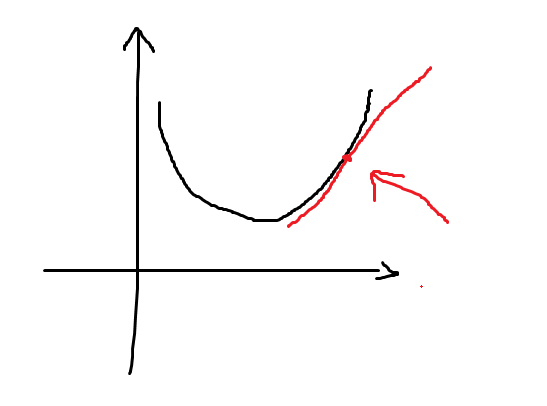箭头所指的地方并是(θ0,J(θ0))，切线则是那里的导数值(d / dθ0) * J(θ0)为正，根据公式θ0 = θ0 − α * (d / dθ0) * J(θ0)其中α 为正数，计算得到的θ0在减小，经过反复的训练最终会逼近图中最低点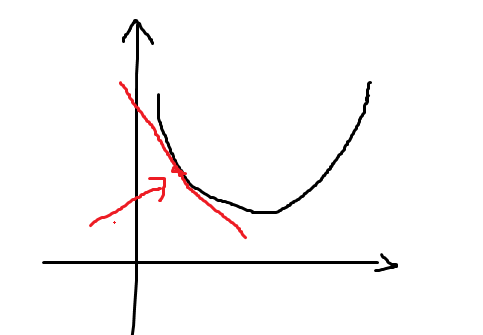同样，切线则是那里的导数值(d / dθ0) * J(θ0)为负，根据公式θ0 = θ0 − α * (d / dθ0) * J(θ0)其中α 为正数，计算得到的θ0在增大，经过反复的训练最终会逼近图中最低点
当在最低点时导数值(d / dθ0) * J(θ0)为0，θ0 = θ0 − α * 0，θ0则不会再变化
由此可见，公式中导数值的意义在于决定θ0向什么方向走，α决定走的步长，但梯度下降法只能达到局部的最优点就像图中的一样只能向最近的最低点移动。


展开全文算法 深度学习 机器学习 python
• 文章目录一、线性回归二、梯度下降法的数学原理三、梯度下降法优化四、Python实现 一、线性回归   关于线性回归的详细介绍可以参见我的上一篇博文《线性回归：最小二乘法优化》。在《线性回归：最小二乘法优化》中...
文章目录一、线性回归二、梯度下降法的数学原理三、梯度下降法优化四、Python实现
一、线性回归
关于线性回归的详细介绍可以参见我的上一篇博文《线性回归：最小二乘法优化》。在《线性回归：最小二乘法优化》中已经说明了线性回归模型建立的关键在于求解：
$(w^*, b^*)=\argmin\sum^{m}_{i=1}{{(f(x_i)-y_i)^2}}$
这里再介绍另一种求解算法：梯度下降法。
二、梯度下降法的数学原理
假设有以下问题：
$w=\argmin f(w)$
通过泰勒一阶展开则有：
$f(w)=f(w_0)+(w-w_0)∇f(w_0)$
图示如下：这里的$w-w_0$表示了移动的步长和方向，那么可以有$w-w_0=\eta\gamma$，$\eta$为实数，表示移动的步长，$\gamma$为一个单位向量，表示步长移动的方向。$f(w_0)+(w-w_0)∇f(w_0)$则是$f(w)$在$w$处的邻近估计，此处要求$\eta$较小，否则将导致估计的精确度降低。
梯度下降算法的目的是让优化函数$f(w)$尽快到达全局最小值，所以便有了第一个条件：
$condition1:f(w)-f(w_0)=\eta\gamma∇f(w_0)≤0$
由于$\eta$是一个大于0的常数，所以可以暂不考虑。那么就需要满足$\eta\gamma∇f(w_0)≤0$并且尽可能小。又有：
$\gamma∇f(w_0)=|\gamma||∇f(w_0)|\cos \alpha$
所以想要使$\eta\gamma∇f(w_0)≤0$并且尽可能小则需要满足条件：
$condition2:\cos \alpha=-1$
换句话说，也就是要求单位向量$\gamma$和$∇f(w_0)$方向完全相反，所以便可以得到以下结论：
$condition3:\gamma=\frac{-∇f(w_0)}{|∇f(w_0)|}$
将$condition3$带入$w-w_0=\eta\gamma$，则有：
$condition4:w-w_0=\eta\frac{-∇f(w_0)}{|∇f(w_0)|}$
由于$\eta$和$|∇f(w_0)|$都是大于0的实数，所以可以合并为新的$\eta^*=\frac{\eta}{|∇f(w_0)|}$，再对等式进行移项便可以得到：
$condition5:w=w_0-\eta∇f(w_0)$
$condition5$便是梯度下降算法中$f(w)$的参数$w$的更新公式。同时这也解释了为什么需要以梯度的反方向来更新权重。
三、梯度下降法优化
下面就用梯度下降算法来对线性回归模型进行优化。这里将线性回归模型的代价函数E写为如下形式（方便运算）：
$E=\frac{1}{2m}\sum^{m}_{i=1}{(f(x_i)-y_i)^2}$
对其求导：
$\frac{\partial E}{\partial w}=\frac{1}{m}\sum^{m}_{i=1}{(wx_i-y_i)\frac{\partial (wx_i-y_i)}{\partial w}}=\frac{1}{m}\sum^{m}_{i=1}{(wx_i-y_i)x_i}$
由前面的$condition5$可以得到权重的更新公式：$w^*=w+\Delta w$，此处的$\Delta w=-\eta∇f(w_0)$。所以最终可以得到权重的更新公式为：
$w^*=w+\frac{\eta}{m}\sum^{m}_{i=1}{(y_i-wx_i)x_i}$
四、Python实现
由之前推导出的更新公式可以实现出以下拟合算法：
def _gradient_descent(self, X, y):
for i in range(max_iter):
delta = y - self._linear_func(X)
self.W += self.eta * sum(delta) / X.shape # 第一列全部为1
self.W[1:] += self.eta * (delta @ X) / X.shape

导入波士顿数据集进行测试：
if __name__ == "__main__":
from sklearn import datasets
from sklearn.model_selection import train_test_split
from sklearn.preprocessing import MinMaxScaler
boston = datasets.load_boston()
X = boston.data
y = boston.target
scaler = MinMaxScaler().fit(X)
X = scaler.transform(X)
X_train, X_test, y_train, y_test = train_test_split(X, y, train_size=0.7, test_size=0.3)
lr = LinearRegression().fit(X_train, y_train)
y_pred = lr.predict(X_test)
from sklearn.metrics import mean_squared_error
print(mean_squared_error(y_test, y_pred))
plt.figure()
plt.plot(range(len(y_test)), y_test)
plt.plot(range(len(y_pred)), y_pred)
plt.legend(["test", "pred"])
plt.show()

均方误差：代价曲线：拟合曲线：展开全文机器学习 python 算法
• 梯度下降法优化目标函数Nowadays we can learn about domains that were usually reserved for academic communities. From Artificial Intelligence to Quantum Physics, we can browse an enormous amount of ...
梯度下降法优化目标函数Nowadays we can learn about domains that were usually reserved for academic communities. From Artificial Intelligence to Quantum Physics, we can browse an enormous amount of information available on the Internet and benefit from it. 如今，我们可以了解通常为学术团体保留的领域。 从人工智能到量子物理学 ，我们可以浏览互联网上大量的信息并从中受益。
However, the availability of information has some drawbacks. We need to be aware of a huge amount of unverified sources, full of factual errors (it’s a topic for the whole different discussion). What’s more, we can get used to getting answers with ease by googling it. As a result, we often take them for granted and use them without a better understanding. 但是，信息的可用性有一些缺点。 我们需要意识到大量未经验证的来源，充满事实错误(这是整个不同讨论的主题)。 而且，我们可以通过谷歌搜索来轻松地轻松获得答案。 结果，我们经常认为它们是理所当然的，并在没有更好的理解的情况下使用它们。
The process of discovering things on our own is an important part of learning. Let’s take part in such an experiment and calculate derivatives behind Gradient Descent algorithm for a Linear Regression. 独自发现事物的过程是学习的重要组成部分。 让我们参加这样的实验，并为线性回归计算梯度下降算法背后的导数。
一点介绍 (A little bit of introduction)
Linear Regression is a statistical method that can be used to model the relationship between variables [1, 2]. It’s described by a line equation: 线性回归是一种统计方法，可用于对变量之间的关系进行建模[1、2]。 它由线方程描述：Line equation (image by Author).We have two parameters Θ₀ and Θ₁ and a variable x. Having data points we can find optimal parameters to fit the line to our data set. 我们有两个参数Θ₀和Θ₁和a   变量x 。 有了数据点，我们可以找到最佳参数以使线适合我们的数据集。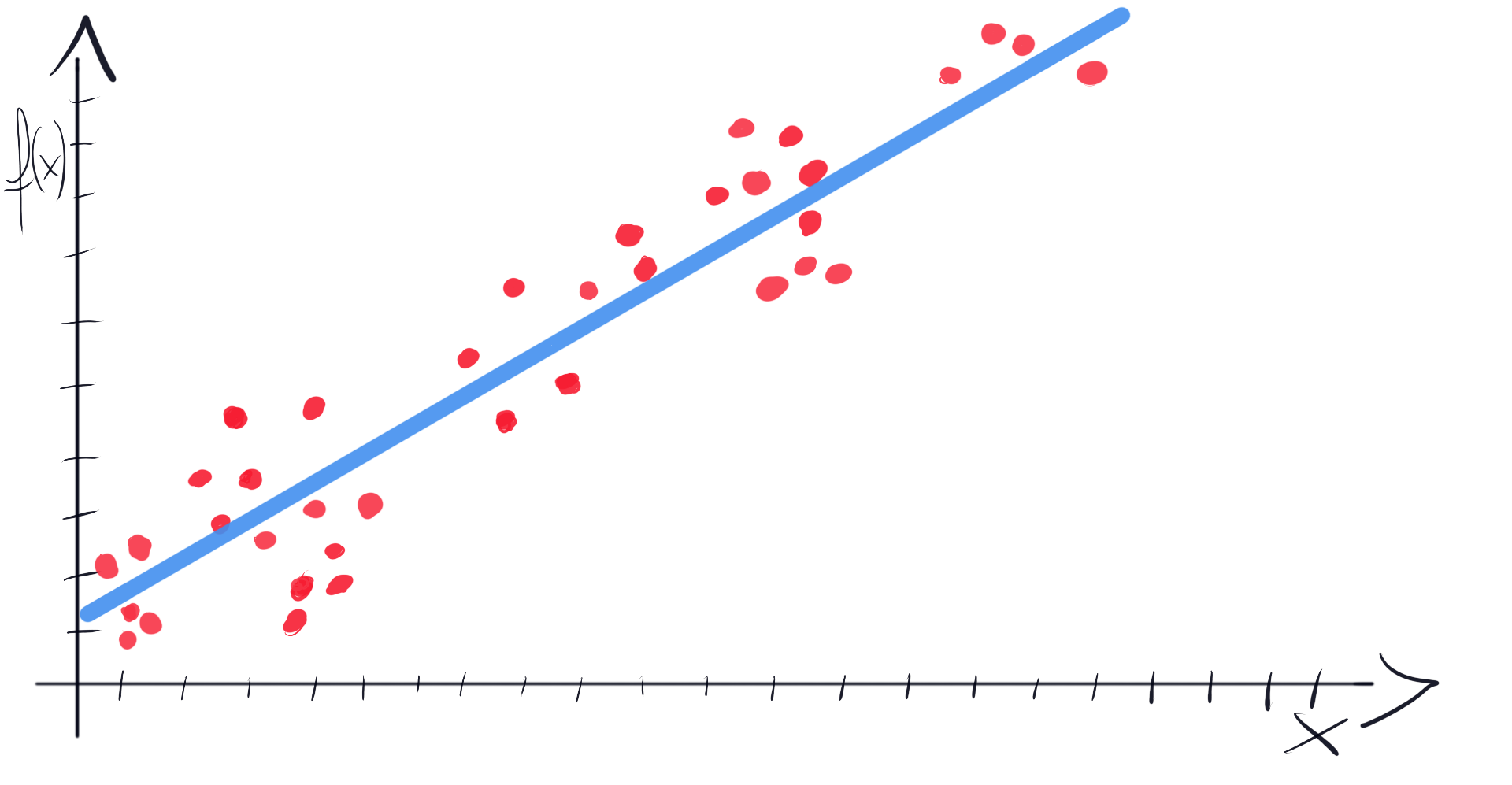Fitting a line to a data set (image by Author).Ok, now the Gradient Descent [2, 3]. It is an iterative algorithm that is widely used in Machine Learning (in many different flavors). We can use it to automatically find optimal parameters of our line. 好的，现在是梯度下降[2，3]。 它是一种迭代算法，已在机器学习中广泛使用(有许多不同的风格)。 我们可以使用它来自动找到生产线的最佳参数。
To do this, we need to optimize an objective function defined by this formula: 为此，我们需要优化由以下公式定义的目标函数：Linear regression objective function (image by Author).In this function, we iterate over each point (xʲ, yʲ) from our data set. Then we calculate the value of a function f for xʲ, and current theta parameters (Θ₀, Θ₁). We take a result and subtract yʲ. Finally, we square it and add it to the sum. 在此函数中，我们迭代数据集中的每个点(xʲ，yʲ) 。 然后我们计算一个函数f x的值，和当前THETA参数(Θ₀，Θ₁)。 我们得到一个结果并减去yʲ 。 最后，我们将其平方并加到总和上。
Then in the Gradient Descent formula (which updates Θ₀ and Θ₁ in each iteration), we can find these mysterious derivatives on the right side of equations: 然后，在“梯度下降”公式(每次迭代中更新Θ₀和Θ₁ )中，我们可以在等式右边找到这些神秘的导数：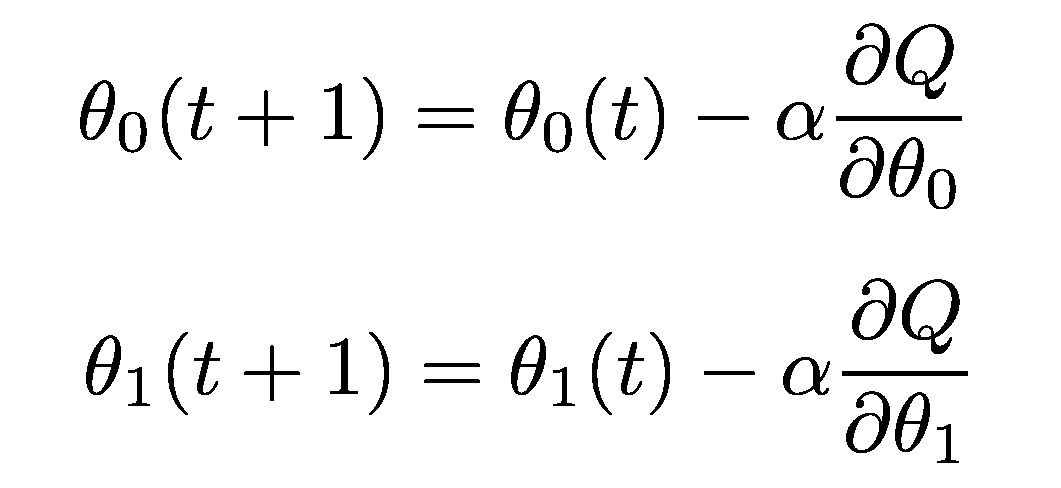Gradient descent formula (image by Author).These are derivatives of the objective function Q(Θ). There are two parameters, so we need to calculate two derivatives, one for each Θ. Let’s move on and calculate them in 3 simple steps. 这些是目标函数Q(Θ)的导数。 有两个参数，因此我们需要计算两个导数，每个Θ一个 。 让我们继续并通过3个简单的步骤计算它们。
步骤1.链式规则 (Step 1. Chain Rule)
Our objective function is a composite function. We can think of it as it has an “outer” function and an “inner” function . To calculate a derivative of a composite function we’ll follow a chain rule: 我们的目标函数是一个复合函数 。 我们可以认为它具有“外部”功能和“内部”功能。 要计算复合函数的导数，我们将遵循一条链规则：Chain rule formula (image by Author).In our case, the “outer” part is about raising everything inside the brackets (“inner function”) to the second power. According to the rule we need to multiply the “outer function” derivative by the derivative of an “inner function”. It looks like this: 在我们的案例中， “外部”部分是关于将方括号内的所有内容( “内部功能” )提升至第二幂。 根据规则，我们需要将“外部函数”导数乘以“内部函数”的导数。 看起来像这样：Applying the chain rule to the objective function (image by Author). 步骤2.功率规则 (Step 2. Power Rule)
The next step is calculating a derivative of a power function . Let’s recall a derivative power rule formula: 下一步是计算幂函数的导数。 让我们回想一下微分幂规则公式：Power rule formula (image by Author).Our “outer function” is simply an expression raised to the second power. So we put 2 before the whole formula and leave the rest as it (2 -1 = 1, and expression raised to the first power is simply that expression). 我们的“外部功能”只是表达为第二力量的表达。 因此，我们将2放在整个公式的前面，其余部分保留为原来的值( 2 -1 = 1 ，升到第一幂的表达式就是该表达式)。
After the second step we have: 第二步之后，我们有：Applying the power rule to the objective function (image by Author).We still need to calculate a derivative of an “inner function” (right side of the formula). Let’s move to the third step. 我们仍然需要计算“内部函数”的导数(公式的右侧)。 让我们转到第三步。
步骤3.常数的导数 (Step 3. The derivative of a constant)
The last rule is the simplest one. It is used to determine a derivative of a constant: 最后一条规则是最简单的规则。 用于确定常数的导数：A derivative of a constant (image by Author).As a constant means, no changes, derivative of a constant is equal to zero . For example f’(4) = 0. 作为常数，没有变化，常数的导数等于零。 例如f'(4)= 0 。
Having all three rules in mind let’s break the “inner function” down: 考虑到所有三个规则，让我们分解一下“内部功能” ：Inner function derivative (image by Author).The tricky part of our Gradient Descent objective function is that x is not a variable. x and y are constants that come from data set points. As we look for optimal parameters of our line, Θ₀ and Θ₁ are variables. That’s why we calculate two derivatives, one with respect to Θ₀ and one with respect to Θ₁. 梯度下降目标函数的棘手部分是x不是变量。 x和y是来自数据设置点的常数。 当我们寻找线的最佳参数时， Θ₀和Θ₁是变量。 这就是为什么我们计算两个导数，一个关于Θ₀ ，一个关于Θ₁。
Let’s start by calculating the derivative with respect to Θ₀. It means that Θ₁ will be treated as a constant. 让我们开始计算关于Θ₀的导数。 这意味着Θ₁将被视为常数。Inner function derivative with respect to Θ₀ (image by Author).You can see that constant parts were set to zero. What happened to Θ₀? As it’s a variable raised to the first power (a¹=a), we applied the power rule. It resulted in Θ₀ raised to the power of zero. When we raise a number to the power of zero, it’s equal to 1 (a⁰=1). And that’s it! Our derivative with respect to Θ₀ is equal to 1. 您会看到常量部分设置为零。 Θ₀怎么了？ 由于它是一个提高到第一幂( a¹= a )的变量，因此我们应用了幂规则。 结果导致Θ₀提高到零的幂。 当我们将数字提高到零的幂时，它等于1( a⁰= 1 )。 就是这样！ 关于Θ₀的导数等于1。
Finally, we have the whole derivative with respect to Θ₀: 最后，我们有了关于Θ₀的整个导数：Objective function derivative with respect to Θ₀ (image by Author).Now it’s time to calculate a derivative with respect to Θ₁. It means that we treat Θ₀ as a constant. 现在是时候来计算相对于Θ₁衍生物。 这意味着我们将Θ₀视为常数。Θ₁θ₁的内函数导数 By analogy to the previous example, Θ₁ was treated as a variable raised to the first power. Then we applied a power rule which reduced Θ₁ to 1. However Θ₁ is multiplied by x, so we end up with derivative equal to x. 与前面的示例类似，将θ₁视为提高到第一幂的变量。 然后我们应用了一个幂规则，将Θ₁减小到1。但是Θ乘以x ，因此最终得到的导数等于x。
The final form of the derivative with respect to Θ₁ looks like this: 关于Θ₁的导数的最终形式如下：Objective function derivative with respect to Θ₁ (image by Author). 完整的梯度下降配方 (Complete Gradient Descent recipe)
We calculated the derivatives needed by the Gradient Descent algorithm! Let’s put them where they belong: 我们计算了梯度下降算法所需的导数！ 让我们将它们放在它们所属的位置：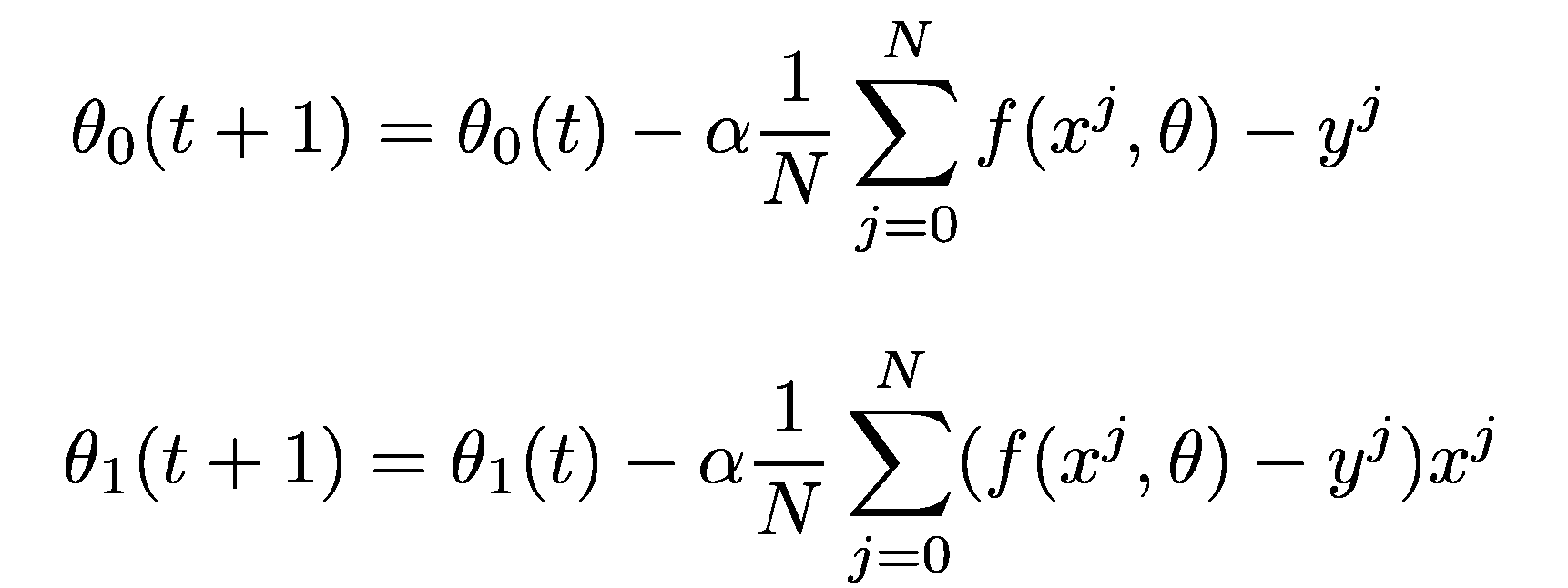Gradient descent formula including objective function’s derivatives (image by Author).By doing this exercise we get a deeper understanding of formula origins. We don’t take it as a magic incantation we found in the old book, but instead, we actively go through the process of analyzing it. We break down the method to smaller pieces and we realize that we can finish calculations by ourselves and put it all together. 通过执行此练习，我们对公式的起源有了更深入的了解。 我们不把它当作在旧书中发现的魔咒，而是积极地进行了分析。 我们将该方法分解为较小的部分，我们意识到我们可以自己完成计算并将其组合在一起。
From time to time grab a pen and paper and solve a problem. You can find an equation or method you already successfully use and try to gain this deeper insight by decomposing it. It will give you a lot of satisfaction and spark your creativity. 时不时地拿笔和纸解决问题。 您可以找到已经成功使用的方程式或方法，并尝试通过分解来获得更深入的了解。 它将给您带来极大的满足感并激发您的创造力。
参考书目： (Bibliography:)
K.A Stroud, Dexter J. Booth, Engineering Mathematics, ISBN: 978–0831133276. KA Stroud，Dexter J. Booth， 工程数学 ，ISBN：978–0831133276。 Joel Grus, Data Science from Scratch, 2nd Edition, ISBN: 978–1492041139 Joel Grus， Scratch的数据科学，第二版 ，ISBN：978–1492041139 Josh Patterson, Adam Gibson, Deep Learning, ISBN: 978–1491914250 Josh Patterson，Adam Gibson， 深度学习 ，ISBN：978–1491914250 翻译自: https://towardsdatascience.com/how-to-differentiate-gradient-descent-objective-function-in-3-simple-steps-b9d58567d387梯度下降法优化目标函数
展开全文• 对于随机梯度下降法，有前面的博客随机梯度下降法实例中可以看出，学习率的选取是很困难的，过大和过小都会出现问题；现在提出一种新方法来选取学习率。 每一轮： learning_rate=0.1*0.99（因为global_step...
对于随机梯度下降法，有前面的博客随机梯度下降法实例中可以看出，学习率的选取是很困难的，过大和过小都会出现问题；现在提出一种新方法来选取学习率。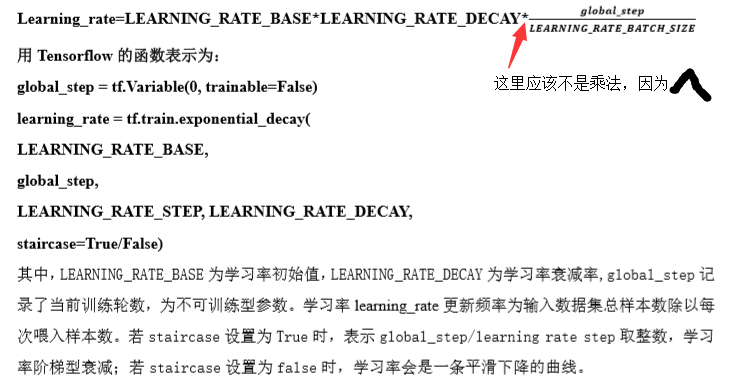每一轮：
learning_rate=0.1*0.99（因为global_step初值给0, 设为不被训练global_step = tf.Variable(0, trainable=False)
代码为：

#coding:utf-8
#设损失函数 loss=(w+1)^2, 令w初值是常数10。反向传播就是求最优w，即求最小loss对应的w值
#使用指数衰减的学习率，在迭代初期得到较高的下降速度，可以在较小的训练轮数下取得更有收敛度。
import tensorflow as tf

LEARNING_RATE_BASE = 0.1 #最初学习率
LEARNING_RATE_DECAY = 0.99 #学习率衰减率
LEARNING_RATE_STEP = 1  #喂入多少轮BATCH_SIZE后，更新一次学习率，一般设为：总样本数/BATCH_SIZE

#运行了几轮BATCH_SIZE的计数器，初值给0, 设为不被训练
global_step = tf.Variable(0, trainable=False)
#定义指数下降学习率
learning_rate = tf.train.exponential_decay(LEARNING_RATE_BASE, global_step, LEARNING_RATE_STEP, LEARNING_RATE_DECAY, staircase=True)
#定义待优化参数，初值给10
w = tf.Variable(tf.constant(5, dtype=tf.float32))
#定义损失函数loss
loss = tf.square(w+1)#tf.square()是对a里的每一个元素求平方
#定义反向传播方法    使用minimize()操作，该操作不仅可以优化更新训练的模型参数，也可以为全局步骤(global_step)计数
train_step = tf.train.GradientDescentOptimizer(learning_rate).minimize(loss, global_step=global_step)
#生成会话，训练40轮
with tf.Session() as sess:
init_op=tf.global_variables_initializer()#初始化
sess.run(init_op)
for i in range(40):#40次
sess.run(train_step)#训练
learning_rate_val = sess.run(learning_rate)#学习率
global_step_val = sess.run(global_step)#计算获取计数器的值
w_val = sess.run(w)#计算权重
loss_val = sess.run(loss)#计算损失函数
#打印相应数据
print "After %s steps: global_step is %f, w is %f, learning rate is %f, loss is %f" % (i, global_step_val, w_val, learning_rate_val, loss_val)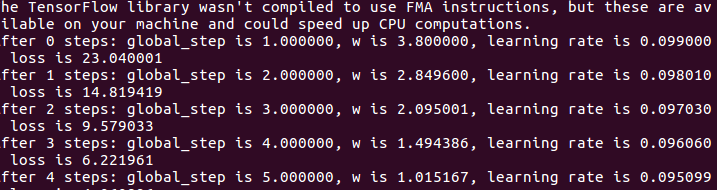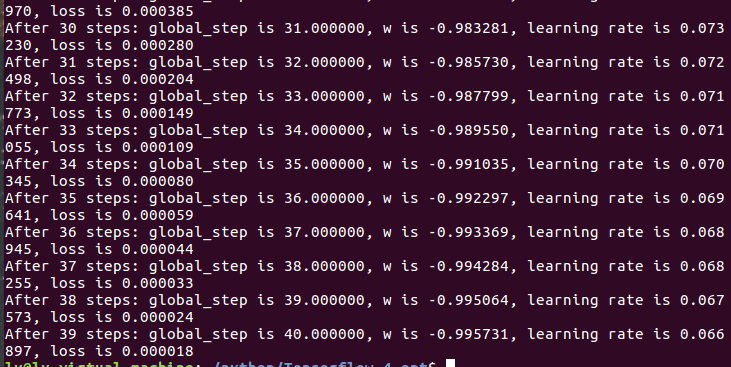global_step
转载于:https://www.cnblogs.com/fcfc940503/p/10961081.html
展开全文• 梯度下降法优化损失函数，其损失函数是由循环产生，再用Spyder运行当中， 其运行的非常慢，感觉进入了死循环损失函数
• 文章目录基于回归模型的协同过滤推荐Baseline：基准预测方法一：随机梯度下降法优化step 1：梯度下降法推导step 2：随机梯度下降step 3：算法实现Step 4: 准确性指标评估方法二：交替最小二乘法优化step 1: 交替最小...python 机器学习 算法 数据分析 深度学习
• 本内容为观看B站，自己手抄代码，不涉及任何利益和机密。 ...seid=12469606868690731782 ...定义一个y_data = x_data*0.1 +0.2的模型函数，经过梯度下降方法，不断优化斜率和截距， #使用numpy生成100个随机点 x
• 图解深度学习-梯度下降法可视化（SGD, Momentum, Adagrad and RMSProp）前言定义了4个基本函数机器学习原理原始的梯度下降算法带动量的梯度下降算法带惯性的梯度下降算法Adagrad 自适应调整的梯度下降RMSPropAdam ...深度学习 图解深度学习 机器学习 人工智能
• 书中并没有给出具体的优化过程，我要来试试试能不能掌握我的梯度下降法啦啦啦啦！！ 梯度下降法 对于优化问题: m i n   f ( x ) (3.1) min\ f(x) \tag{3.1} m i n   f ( x ) ( 3 . 1 )     梯度 ...
• #定义一个梯度下降法来进行训练的优化器 optimizer=tf .train .GradientDescentOptimizer ( 0.2 ) #最小化代价函数 train=optimizer .minimize (loss) init=tf .global _variables_initializer() with tf ....
• 文章目录线性回归简介线性回归应用场景什么是线性回归定义与...求f`(x)3.2 y=ln(sinx), 求dy/dx矩阵（向量）求导 [了解]线性回归的损失和优化损失函数优化算法正规方程什么是正规方程正规方程求解举例正规方程的推...机器学习 人工智能 深度学习 python 算法...